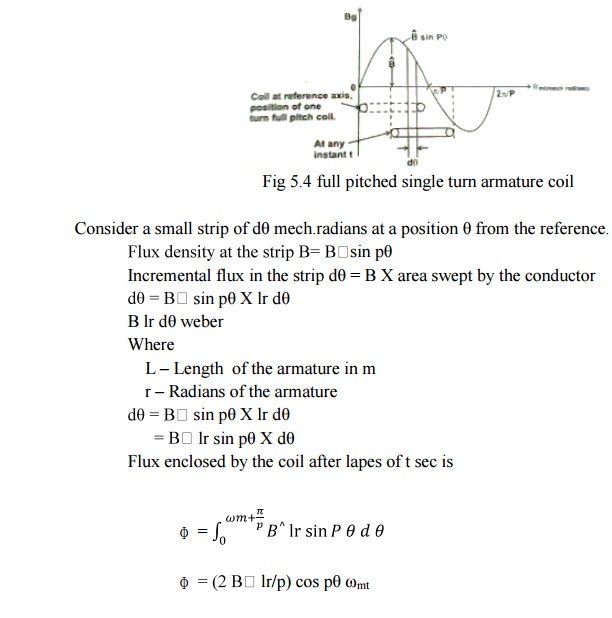Home | | Special Electrical Machines | EMF Equation of BLPM Sine Wave Motor

# EMF Equation of BLPM Sine Wave Motor

1. Flux density distribution 2. EMF Equation of an ideal BLPM sine wave motor 3. EMF equation of practical BLPM sine wave motor

EMF EQUATION OF BLPM SINE WAVE MOTOR

1. Flux density distribution

Flux density can be expressed as B=||B|| sinθ or B  |cospθ  or B sin(Pθ+a) or cos (ρθ+α),2p=p,(i.e)p-no of pole pairs depending upon the position of the reference axis as shown in fig6.3Consider a full pitched single turn armature coil as shown in fig 5.4.Let the rotor be revolving with a uniform angular velocity of m mech.rad/sec.

At time t = 0, let the axis of the single turn coil be along the polar axis.2. EMF Equation of an ideal BLPM sine wave motor

As per faradays law of electromagnetic induction, emf induction in the single turn coil.

e = -N d ɸ /dtlet the armature winding be such that all turns of the phase are concentrated full pitched and located with respect to pole axis in the same manner.

Let Tph be the number of turns connected in series per phase. Then the algebraic addition of the emfs of the individual turns gives the emf induced per phase as all the emf are equal and in phase.3. EMF equation of practical BLPM sine wave motor

In a practical BLPM sine wave motor at the time of design it is taken care to have the flux density is sinusoidal distributed and rotor rotates with uniform angular velocity. However armature winding consists of short chorded coils properly distributed over a set of slot.

These aspect reduce the magnitude of E ph of an ideal winding by a factor Kw1 which is known as the winding factor the fundamental component of flux.Study Material, Lecturing Notes, Assignment, Reference, Wiki description explanation, brief detail
Special Electrical Machines : Permanent Magnet Synchronous Motor : EMF Equation of BLPM Sine Wave Motor |